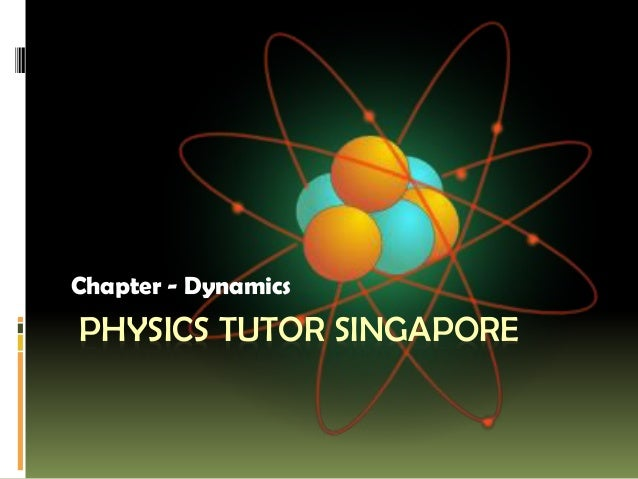Successfully reported this slideshow.Upcoming SlideShare
×

# Physics Tutor Singapore

265 views

Published on

Our Physics Syllabus Chapter - Dynamics, solve problems for a static point mass under the action of 3 forces for 2-dimensional cases (a graphical method would suffice).

Published in: Education
• Full Name
Comment goes here.

Are you sure you want to Yes No• Be the first to comment

• Be the first to like this

### Physics Tutor Singapore

1. 1. PHYSICS TUTOR SINGAPORE Chapter - Dynamics
2. 2. Dynamics Content • Balanced and unbalanced forces • Free-body diagram
3. 3. • Friction Learning Outcomes Candidates should be able to:
4. 4. (a) apply Newton's Laws to: (iii) identify action-reaction pairs acting on two interacting bodies (stating of Newton's Laws is not required) (i) describe the effect of balanced and unbalanced forces on a body (ii) describe the ways in which a force may change the motion of a body
5. 5. (b) identify forces acting on an object and draw free body diagram(s) representing the forces acting on the object (for cases involving forces acting in at most 2 dimensions)
6. 6. (c) solve problems for a static point mass under the action of 3 forces for 2- dimensional cases (a graphical method would suffice) (d) recall and apply the relationship resultant force = mass × acceleration to new situations or to solve related problems
7. 7. (e) explain the effects of friction on the motion of a body.
8. 8. Contact us  (Call/SMS) - 81356556  Website - www.sgphysicstuition.com